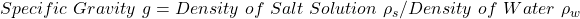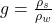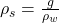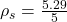## A concentrated salt solution has a mass of 5.29 g for a 5.00 mL sample. What is the specific gravity of this solution

Question

A concentrated salt solution has a mass of 5.29 g for a 5.00 mL sample. What is the specific gravity of this solution

in progress 0
6 months 2021-07-26T12:37:52+00:00 1 Answers 35 views 0

## Answers ( )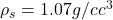Explanation:

From the question we are told that:

Mass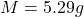Volume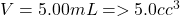Generally the equation for Specific Gravity momentum is mathematically given by